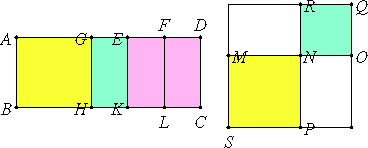# Proposition 59

If an area is contained by a rational straight line and the sixth binomial, then the side of the area is the irrational straight line called the side of the sum of two medial areas.

Let the area ABCD be contained by the rational straight line AB and the sixth binomial AD, divided into its terms at E, so that AE is the greater term.

I say that the side of AC is the side of the sum of two medial areas.

Make the same construction as shown before.

It is then clear that MO is the side of AC, and that MN is incommensurable in square with NO.X.21

Now, since EA is incommensurable in length with AB, therefore EA and AB are rational straight lines commensurable in square only, therefore AK, that is, the sum of the squares on MN and NO, is medial.

Again, since ED is incommensurable in length with AB, therefore FE is also incommensurable with EK. Therefore FE and EK are rational straight lines commensurable in square only. Therefore EL, that is, MR, that is, the rectangle MN by NO, is medial.

Since AE is incommensurable with EF, therefore AK is also incommensurable with EL.

But AK is the sum of the squares on MN and NO, and EL is the rectangle MN by NO, therefore the sum of the squares on MN and NO is incommensurable with the rectangle MN by NO. And each of them is medial, and MN and NO are incommensurable in square.

X.41

Therefore MO is the side of the sum of two medial areas, and is the side of AC.

Therefore, if an area is contained by a rational straight line and the sixth binomial, then the side of the area is the irrational straight line called the side of the sum of two medial areas.

Q.E.D.

## Guide

This proposition is used in X.72.